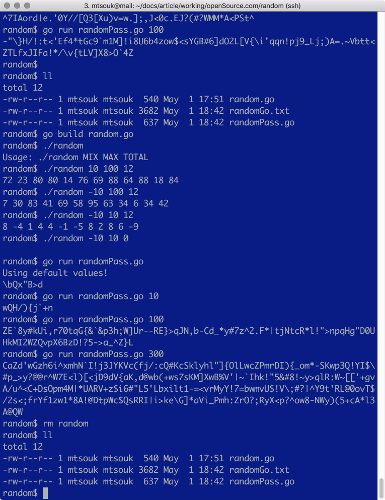# Creating random, secure passwords in Go

## Go's random number generator is a great way to generate difficult-to-guess passwords.Image credits :
geralt, via Pixabay. CC0.

## Subscribe now

Get the highlights in your inbox every week.

You can use the random number generator provided by the Go programming language to generate difficult-to-guess passwords comprised of ASCII characters. Although the code presented in this article is easy to read, it's best if you already know the basics of Go to understand it. If you're new to the programming language, take the Tour of Go to learn more, then come back here.

Before we get into the utilities and the code, take a look at this subset of the ASCII table as found in the output of the `man ascii` command:

``` 30 40 50 60 70 80 90 100 110 120
---------------------------------
0:    (  2  <  F  P  Z  d   n   x
1:    )  3  =  G  Q  [  e   o   y
2:    *  4  >  H  R  \  f   p   z
3: !  +  5  ?  I  S  ]  g   q   {
4: "  ,  6  @  J  T  ^  h   r   |
5: #  -  7  A  K  U  _  i   s   }
6: \$  .  8  B  L  V  `  j   t   ~
7: %  /  9  C  M  W  a  k   u  DEL
8: &  0  :  D  N  X  b  l   v
9: '  1  ;  E  O  Y  c  m   w```

The printable ASCII characters' decimal values range from 33 through 126; no other ASCII values are suitable for inclusion in passwords. Therefore, the utilities presented in this article will produce ASCII characters in that range.

## Creating random integers

The first utility is named `random.go`, and it generates a specified number of random integers that reside in a given range. The most important part of `random.go` is this function:

```func random(min, max int) int {
return rand.Intn(max-min) + min
}```

This function generates random integers that belong to a given range using the `rand.Intn()` Go function. Note that `rand.Intn()` returns a non-negative random number that belongs to `[0,n)`; the function will panic if its argument is a negative number. The panic message will be `panic: invalid argument to Intn`. You can find the documentation of the `math/rand` package at math/rand documentation.

The `random.go` utility accepts three command-line parameters: the minimum value of the generated integers, the maximum value, and the number of integers that will be generated.

Compiling and executing `random.go` will create this kind of output:

```\$ go build random.go
\$ ./random
Usage: ./random MIX MAX TOTAL
\$ ./random 1 3 10
2 2 1 2 2 1 1 2 2 1```

If you wish to generate more secure random numbers in Go, use the `crypto/rand` package of the Go library.

The second utility, `randomPass.go`, generates the random passwords. `randomPass.go` uses the `random()` function to generate random numbers that will convert to ASCII characters using the following Go code:

```for {
myRand := random(MIN, MAX)
newChar := string(startChar + byte(myRand))
fmt.Print(newChar)
if i == LENGTH {
break
}
i++
}```

The value of MIN is 0 and the value of MAX is 94, whereas the value of `startChar` is !, which is the first printable character in the ASCII table (with the decimal ASCII code of 33). Therefore, all ASCII characters that will be generated are located after ! and before the ~ character, which has a decimal ASCII code of 126.

So, each random number that is generated is bigger than MIN, smaller than MAX, and converted into an ASCII character. The process keeps going until the generated password has the desired length.

The `randomPass.go` utility accepts a single (optional) command-line parameter that defines the length of the generated password. Its default value is eight, which is a pretty common password length. Executing `randomPass.go` will generate the following kind of output:

```\$ go run randomPass.go 1
Z
\$ go run randomPass.go 10
#Cw^a#IwkT
\$ go run randomPass.go
Using default values!
[PP8@'Ci```

One last detail: Don't forget to call `rand.Seed()` with a seed value in order to initialize the random number generator. If you use the same seed value all the time, the random number generator will create the same sequence of random integers.You can find both `random.go` and `randomPass.go` at GitHub. You can also execute it at play.golang.org.